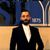# Attribute table statistics using arcpy (for polyline)

768
4
03-30-2021 03:22 AM
Labels (3)New Contributor III

hello, I have a polyline shapefile, and I need to calculate the mean value of one column of its attribute table using arcpy.

Any suggestion?

Tags (4)
1 Solution

Accepted SolutionsbyMVP Esteemed Contributor
``````import numpy as np
from arcpy.da import SearchCursor
values = np.mean([row for row in SearchCursor(fc, "Shape_Length")])``````

An equivalent but slower method, if there are no nulls (an extra hoop to jump through is necessary if there are nulls)

... sort of retired...
4 RepliesbyMVP Esteemed Contributor

or is it something different you are looking for?

... sort of retired...byMVP Esteemed Contributor

or perhaps

``````import numpy as np
from arcpy.da import TableToNumPyArray
fc = r"C:\Git_Dan\npgeom_bak\Project_npg\npgeom.gdb\Ontario_singlepart"
arr = TableToNumPyArray(fc, "Shape_Length", skip_nulls=True)

np.mean(arr["Shape_Length"])
84684.37997765756``````

... sort of retired...New Contributor III

I will try this one.

Thanks a lot.byMVP Esteemed Contributor
``````import numpy as np
from arcpy.da import SearchCursor
values = np.mean([row for row in SearchCursor(fc, "Shape_Length")])``````

An equivalent but slower method, if there are no nulls (an extra hoop to jump through is necessary if there are nulls)

... sort of retired...